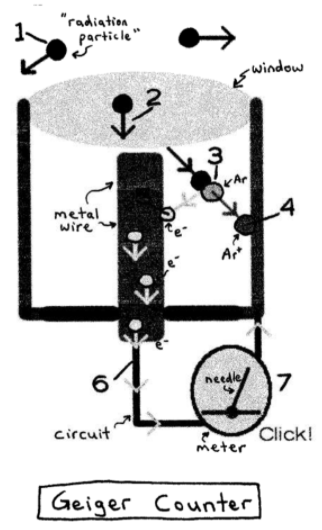# S19E3 - Half-Life and the Detection of Radioactivity

Today we're going to talk about the half-lives of nuclear reactions and radioactive decay processes.

We'll also discuss the Geiger counter and how it's used to detect radioactivity.

----------

## Half-Life

Half-Life (t½)  =  the time required for the number of nuclides to reach half the original value.

Assuming first-order kinetics (see Section 12), the equation for the half-life is as follows:

t½  =  ln 2 / k  =  0.693 / k  ,  where k is the rate constant.The half-lives of radioactive isotopes (nuclides) can vary tremendously, ranging from 109 years to 10-4 seconds.

Um... that's a range of 1,000,000,000 years to only 0.0001 seconds!

----------

ex:  Consider technetium-99, or 99Tc.  The rate constant for its radioactive decay is 1.16 x 10-1 hr-1.  Calculate the half-life of this nuclide.
_________

➞  we'll need the half-life equation:

t½  =  0.693 / k

t½  =  0.693 / 0.116 hr-1

t½  =  5.98 hrSo, it takes almost 6 hours for a sample of Tc-99 to decrease to half the number of original nuclides.

==========

Radioactive decay processes generate high energy particles such as alpha-particles, beta-particles, and gamma-rays.

These particles can produce ions when traveling through matter.

A geiger counter takes advantage of this...

----------

### How Does a Geiger Counter Work?

Geiger counter  =  measures radioactivity by "capturing" particles through a window opening in the counter.

Once inside, these radioactive particles ionize Ar(g) to Ar+(g), and it's the amount of Ar+(g) that gets measured.

Here's the 7 steps involved...

2.  Some radiation enters the window.

3.  When radiation (α-particle, etc.) collides with Ar(g) in the tube, it causes:

Ar(g)  →  Ar+(g)  +  e-4.  The positively-charged Ar+ ions are attracted to the outside of the tube.

5.  The electrons (e- 's) are attracted to a wire running down the middle of the tube.

6.  Many electrons travel down the wire, making a "burst" of current in a circuit connected to it.

7.  Electrons make the meter's needle deflect, and the loudspeaker makes a "click" every time particles are detected.

==========

Next up in SECTION 19 - The Nucleus and Nuclear Chemistry,

Hope you stick around!...